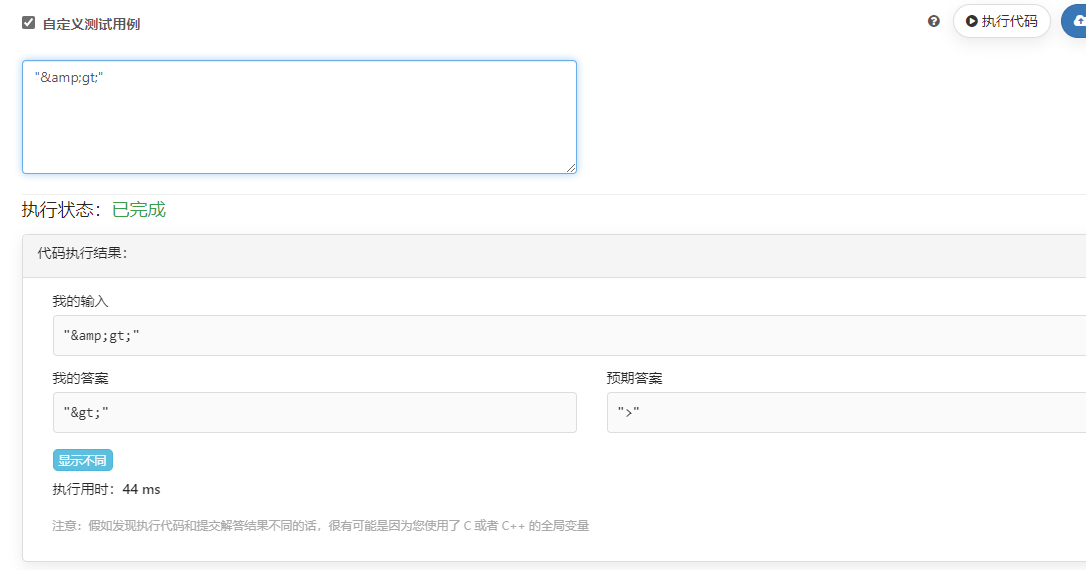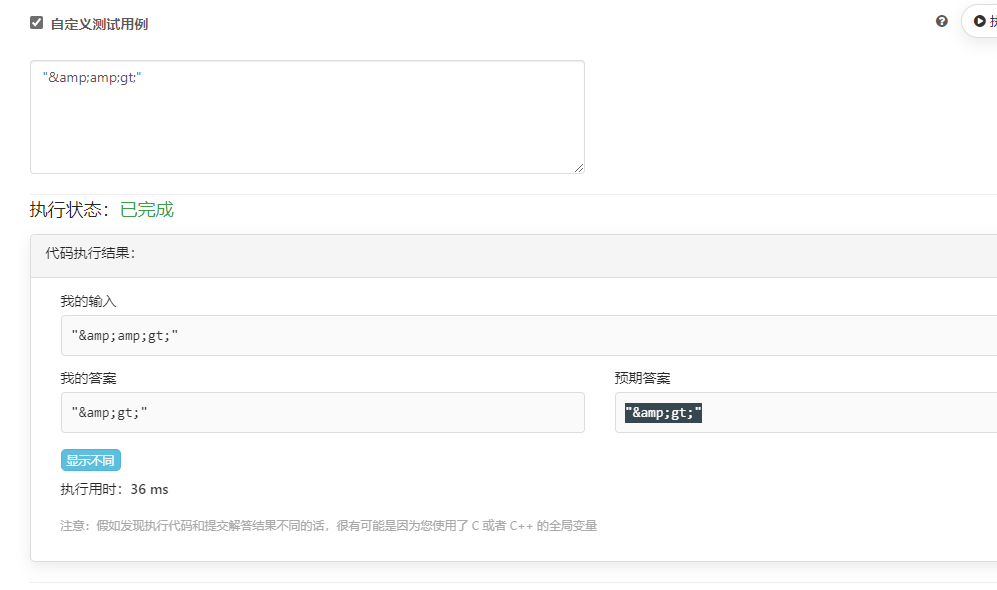🏆 第 184 场力扣周赛

python 题解 https://leetcode-cn.com/circle/article/oCBsqp/

## 5380.数组中的字符串匹配

class Solution:
def stringMatching(self, words: List[str]) -> List[str]:
ans = []
for i in range(len(words)):
for j in range(len(words)):
if i == j: continue
if words[i] in words[j]:
ans.append(words[i])
break
return ans


## 5381.查询带键的排列

class Solution:
def processQueries(self, queries: List[int], m: int) -> List[int]:
m = min(m, max(queries))
p = [i+1 for i in range(m)]
ans = []
for x in queries:
i = p.index(x)
ans.append(i)
x = p.pop(i)
p.insert(0, x)
return ans


## 5382.HTML 实体解析器

class Solution:
def entityParser(self, text: str) -> str:
text = text.replace('&quot;', '"')
text = text.replace('&apos;', '\'')
text = text.replace('&amp;', '&')
text = text.replace('&gt;', '>')
text = text.replace('&lt;', '<')
text = text.replace('&frasl;', '/')
return text


class Solution:
def entityParser(self, text: str) -> str:
text = text.replace('&quot;', '"')
text = text.replace('&apos;', '\'')
text = text.replace('&amp;', '&')
text = text.replace('&gt;', '>')
text = text.replace('&lt;', '<')
text = text.replace('&frasl;', '/')
return text## 5383.给 N x 3 网格图涂色的方案数

A 开头有：
ABA ABC ACA ACB
B 开头有：
BAB BAC BCA BCB
C 开头有：
CAB CAC CBA CBC

ABA 模式有 6 种：ABA ACA BAB BCB CAC CBC
ABC 模式有 6 种：ABC ACB BAC BCA CAB CBA

第一层   ABA   ABA   ABA   ABA   ABA
|||   |||   |||   |||   |||



第一层   ABC   ABC   ABC   ABC
|||   |||   |||   |||



MOD = 1000000007
aba2 = (aba * 3 + abc * 2) % MOD
abc2 = (aba * 2 + abc * 2) % MOD


aba 和 abc 初始都为 6

class Solution:
def numOfWays(self, n: int) -> int:
aba = 6
abc = 6
MOD = 1000000007
for _ in range(n-1):
aba2 = (aba * 3 + abc * 2) % MOD
abc2 = (aba * 2 + abc * 2) % MOD
aba, abc = aba2, abc2
return (aba + abc) % MOD

25

## B: 查询带键的排列

1. 扫描数组确定数字所在下标
2. 删除该数字
3. 将该数字插入数组的头部

## C: HTML 实体解析器

1. 能被替换的字符串都是以 '&' 开头的
2. 顺序扫描原串 text，遇到非 '&' 直接保留
3. 遇到是 '&' 则判断后面连续的 4 ~ 7 个字符组成的子串是否为需要替换的字符串（最短的是 ">" 最长的是 "⁄" 长度分别为 4 和 7）
4. 替换完后，扫描 text 的指针直接跳跃到替换后的字符下标；因此该指针不会回溯移动

## D: 给 N x 3 网格图涂色的方案数

1. dp[row][col] 表示合法构造了前 row 行且第 row 的配色为 col 的总方案数
2. dp[row][col] = sum(dp[row-1][col_1])，其中 col 和 col_1 是不冲突的两种配色
3. 最终答案 result = sum(dp[n-1][col])，col 来自那合法的 12 种方案

## 关注微信公众号查看代码10

class Solution:
def numOfWays(self, n: int) -> int:
same, diff = 6, 6    # 代表初始时第一行两类各6种
for k in range(1,n):
same, diff = same * 3 + diff * 2, same * 2 + diff * 2
return (same + diff) % (10 ** 9 + 7)

# 所有排列其实只有两种：
#     第一类：第一格和第三格相同的:
#     第一类：第一格和第三格不同的
# 具体是什么颜色不重要，只要区分这两类就行了
# 在已知上一行时，下一行的演化是：
#     如果上一行是第一类：共5种可能，其中3种一类，2种二类
#     如果上一行是第二类：共4种可能，其中2种一类，2种二类
#     然后更新这一行每一类的个数，一直迭代下去就行了

3

#### 5380. 数组中的字符串匹配

O(n^2) 暴力枚举，具体思路见注释。介绍一下 string 的 find 函数。

• std::string::find 函数。接收一个字符串，返回该字符串第一次出现的位置。
• 如果没有找到，返回 std::string::npos。
class Solution {
public:
vector<string> stringMatching(vector<string>& words) {
vector<string> res;
//枚举每个字符串作为子串。
for(int i = 0; i < words.size(); i++) {
//对于每个子串，枚举所有字符串作为其母串。
for(int j = 0; j < words.size(); j++) {
//母串和子串不能是同一个字符串，所以需要 i != j
//检查words[i] 是否是 words[j] 的子串。
if(i != j && words[j].find(words[i]) != std::string::npos) {
res.push_back(words[i]);
break;
}
}
}
return res;
}
};


#### 5381. 查询带键的排列

O(m*q) 暴力枚举。使用链表模拟即可，具体思路见注释。简单介绍下 std::list 的 insert 和 erase 函数。

• std::list::insert 可以接受一个std::list::iterator iter和一个待插入元素 p。该函数会将 p 插入到 iter 之前。
• std::list::erase 可以接受一个std::list::iterator iter。该函数会删除 iter 指向的结点。
class Solution {
public:
vector<int> processQueries(vector<int>& queries, int m) {
list<int> dataList;
//初始化排列。
for(int i = 1; i <= m; i++) {
dataList.push_back(i);
}
vector<int> res;
//处理每个请求
for(auto v : queries) {
auto it = dataList.begin();
int ans = 0;
//O(m)的查找当前询问的位置。
for(auto it = dataList.begin(); it != dataList.end(); ++it, ++ans) {
// 找到了
if(*it == v) {
res.push_back(ans); //记录答案
//在链表的头部插入该元素。
dataList.insert(dataList.begin(), *it);
//删除旧的元素。
dataList.erase(it);
break;
}
}
}
return res;
}
};


#### 遍历 text

class Solution {
public:
string entityParser(string text) {
string res;
unordered_map<string, string> dict; //将特殊字符和字符实体放入 dict 中。
dict["&quot;"] = "\"";
dict["&apos;"] = "'";
dict["&amp;"] = "&";
dict["&gt;"] = ">";
dict["&lt;"] = "<";
dict["&frasl;"] = "/";

for(int i = 0, n = text.size(); i < n; ) {//遍历text
bool match = false;
//判断以text[i]为起点的字符串是否是特殊字符
for(auto p = dict.cbegin(); p != dict.cend(); p++) {
//匹配上了
if(text.substr(i, p->first.size()) == p->first) {
res += p->second;
i += p->first.size(); //i 后移 p->first.size()
match = true; //标记已匹配
break;
}
}
if(match) {
continue;
}
res += text[i++]; //未匹配上，将 text[i] 放入 res。i 后移一位。
}
return res;
}
};


#### 递归

dp[n][sc] += dp[n-1][psc] (0 <= psc < 27)。

class Solution {
public:
const int mod = 1000000007;
int dp = {0};
//根据每个格子的颜色获取状态码
int sc(int i, int j, int k) {
return i + j*3 + k *9;
}
//根据状态码获取每个格子的颜色
void cs(int statusCode, int &a, int &b, int &c) {
a = statusCode % 3;
b = (statusCode - a)%9/3;
c = statusCode/9;
}

int numOfWays(int n) {
初始化 n = 1 的方案数。
for(int i = 0; i <= 2; i++) {
for(int j = 0; j <= 2; j++) {
for(int k = 0; k <= 2; k++) {
if(i != j && j != k) {
dp[sc(i,j,k)] = 1;
}
}
}
}
//枚举行数
for(int level = 2; level <= n; level++) {
//枚举前一行的染色方案
for(int pre = 0; pre < 27; ++pre) {
int a, b, c;
cs(pre, a, b, c);
if(a == b || b == c) {
continue;
}
//枚举当前行的染色方案
for(int i = 0; i <= 2; i++) {
if(i == a) { continue; } //检查是否冲突
for(int j = 0; j <= 2; j++) {
if(i == j || j == b) { continue; } //检查是否冲突
for(int k = 0; k <= 2; k++) {
if(j == k || k == c) { continue; } //检查是否冲突
(dp[level][sc(i,j,k)] += dp[level-1][pre]) %= mod; //当前染色方案可行，累加方案数。
}
}
}
}
}
int ans = 0;
for(int i = 0; i < 27; i++) {
(ans += dp[n][i]) %= mod; //计算总的方案数。
}
return ans;
}
};

3

2

1

1

1

• ABA
本行能涂：
BCB
BAB
BAC
CAB
CAC
以上共5种方案，其中ABC类的2种，ABA类的3种
同理，上一行是ABC类的：
• ABC
本行能涂：
BAB
BCA
BCB
CAB
以上共5种方案，其中ABC类2种，ABA类2种。

1